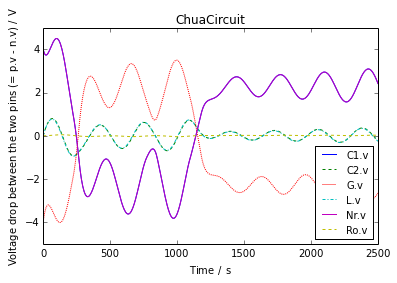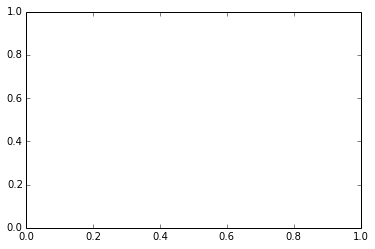# ModelicaRes Tutorial¶

This tutorial shows how to use ModelicaRes to run Modelica model experiments and analyze the results.

Before we get started, we'll load some standard modules and establish settings for this IPython notebook.

In :
import numpy as np
import matplotlib.pyplot as plt
import pandas; pandas.set_option('display.max_rows', 5)
%matplotlib inline
%precision 4

Out:
u'%.4f'

## Running model experiments¶

ModelicaRes can help run sets of model simulations or linearizations. The usage is based on special context managers called simulators. For example, to run a dymosim executable model several times with different settings:

In :
from modelicares.exps.simulators import dymosim

model = "ChuaCircuit/dymosim"
with dymosim(results_dir='ChuaCircuit', StopTime=2500) as simulator:
simulator.run(model, {'L.L': 15})
simulator.run(model, {'L.L': 18})
simulator.run(model, {'L.L': 21})


This simulates the model three times with the desired parameters and places the results in three subfolders. Each folder contains an initialization file, a final values file, full results (*.mat), and a log file. In the base folder there is a tab-separated values file (runs.tsv) with a summary of the simulations.

Currently, two other simulators are available: dymola_script to write a Dymola-formatted simulation script (*.mos) to perform the same actions as above and fmi to run FMUs in a similar manner via PyFMI. With the dymosim and fmi simulators, one can continue a previous simulation by using continue_run() instead of run().

In order to easily build a large set of simulations with varying parameters, we can use the design of experiments module (doe). For example, to run a full-factorial study:

In :
from modelicares import doe

with dymosim(results_dir='ChuaCircuit', StopTime=2500) as simulator:
for params in doe.fullfact({'C1.C': [8, 10], 'L.L': [18, 20]}):
simulator.run(model, params)


This runs the model four times and places the results in four subfolders. In the "Initial values & parameters" column of the summary file (runs.tsv) we see:

• C1(C=8), L(L=18)
• C1(C=10), L(L=18)
• C1(C=8), L(L=20)
• C1(C=10), L(L=20)

## Analyzing results¶

### Simulation results¶

First, we'll load a simulation result of Modelica.Electrical.Analog.Examples.ChuaCircuit:

In :
from modelicares import SimRes
sim = SimRes('ChuaCircuit.mat')


Now we'll explore the simulation results using the methods in SimRes. To get the start and stop times of the simulation, we can look up the initial and final values of time:

In :
sim['Time'].IV

Out:
0 s
In :
sim['Time'].FV

Out:
2500 s

There are many other properties. For example,

In :
Lv = sim['L.v']
dict(description=Lv.description,
display_unit=Lv.display_unit,
maximum=Lv.max,
mean=Lv.mean,
minimum=Lv.min,
rms=Lv.RMS)

Out:
{'description': u'Voltage drop between the two pins (= p.v - n.v)',
'display_unit': V,
'maximum': 0.773441 V,
'mean': 0.0147338 V,
'minimum': -0.945017 V,
'rms': 0.366374 V}

The display unit was loaded from the result, but we can change it:

In :
Lv.display_unit = 'mV'
dict(maximum=Lv.max,
mean=Lv.mean,
minimum=Lv.min,
rms=Lv.RMS)

Out:
{'maximum': 773.441 mV,
'mean': 14.7338 mV,
'minimum': -945.017 mV,
'rms': 366.374 mV}

To retrieve the values of a variable, use values():

In :
from natu.units import s  # The unit second
Lv.values(t=(20*s,))

Out:
[   0.      109.235   210.835   304.6235  390.4008] mV

Here we've only included the first 20 seconds, but all of the values are returned by default. To return the sample times, use times():

In :
Lv.times(t=(20*s,))

Out:
[  0.   5.  10.  15.  20.] s

These values have been extracted directly, but it's also possible to interpolate at a specified time:

In :
Lv.values(t=2.5*s)

Out:
54.6175 mV

or at a specified list of times:

In :
Lv.values(t=[2.5*s, 7.5*s, 12.5*s, 17.5*s]) # TODO: return as array

Out:
[54.6175 mV, 160.035 mV, 257.729 mV, 347.512 mV]

or to return only every nth sample (here, n = 100):

In :
t = (None, None, 100)
zip(Lv.times(t), Lv.values(t))

Out:
[(0 s, 0 mV),
(480 s, 234.305 mV),
(960 s, -522.736 mV),
(1440 s, 127.059 mV),
(1940 s, -138.497 mV),
(2440 s, 138.457 mV)]
In :
x=sim['Ro.R']


To check if a variable is included in the results, we can use Python's in operator.

In :
'L.v' in sim

Out:
True

To see how many variables are in a simulation, use Python's len() function:

In :
len(sim)

Out:
62

To search for variable names, use names() with wildcards:

In :
sim.find('L*v')

Out:
['L.n.v', 'L.p.v', 'L.v']

Regular expressions can also be used:

In :
voltages = sim.find('^[^.]*.v\$', re=True)
voltages

Out:
['C1.v', 'C2.v', 'G.v', 'L.v', 'Nr.v', 'Ro.v']

We can access information about all these variables at once:

In :
sim(voltages).IV

Out:
[4 V, 0 V, -4 V, 0 mV, 4 V, 0 V]

Notice that we used parentheses; sim[] only accepts one variable and would have produced an error. Also notice that millivolts are used to display the value of L.v, but the other display units have not changed.

We can plot these voltages using plot():

In :
sim.plot(voltages);The title, axis labels, and legend entries were generated automatically, but they can be customized using other plot arguments or functions from matplotlib.pyplot. The abscissa can be any variable, not just time. Here, we use current:

In :
ax, __ = sim.plot(ynames1='L.v', ylabel1="Voltage",
xname='L.i',  xlabel="Current",
title="Chua circuit\n"
"Current through and voltage across the inductor")

# Mark the start and stop points.
def mark(time, text):
i = sim['L.i'].values(time)
v = sim['L.v'].values(time)
plt.plot(i, v, 'bo')
ax.annotate(text, xy=(i, v), xytext=(0, -4), ha='center', va='top',
textcoords='offset points')
mark(0, "Start")
mark(2500, "Stop")

---------------------------------------------------------------------------
ValueError                                Traceback (most recent call last)
<ipython-input-21-fa06e2c68888> in <module>()
1 ax, __ = sim.plot(ynames1='L.v', ylabel1="Voltage",
2                   xname='L.i',  xlabel="Current",
----> 3                   title="Chua circuit\n"
4                         "Current through and voltage across the inductor")
5

/usr/local/lib/python2.7/dist-packages/ModelicaRes-0.12.2_97_g5fd3eaa_dirty-py2.7.egg/modelicares/simres.pyc in plot(self, ynames1, ylabel1, f1, legends1, leg1_kwargs, ax1, ynames2, ylabel2, f2, legends2, leg2_kwargs, ax2, xname, xlabel, title, label, incl_prefix, suffix, use_paren, **kwargs)
1427                 util.plot(y2, y2times, ax2, label=legends2, **kwargs)
1428             else:
-> 1429                 util.plot(y1, y1times, ax1, label=legends1, **kwargs)
1430         elif ynames2:
1431             util.plot(y2, y2times, ax2, label=legends2, **kwargs)

/usr/local/lib/python2.7/dist-packages/ModelicaRes-0.12.2_97_g5fd3eaa_dirty-py2.7.egg/modelicares/util.pyc in plot(y, x, ax, label, color, marker, dashes, **kwargs)
899                          color=next(color), marker=next(marker),
900                          dashes=next(dashes), **kwargs)
--> 901                  for i, (xi, yi) in enumerate(zip(x, y))]
902
903     return plots

/usr/lib/pymodules/python2.7/matplotlib/axes.pyc in plot(self, *args, **kwargs)
4135         lines = []
4136
-> 4137         for line in self._get_lines(*args, **kwargs):
4139             lines.append(line)

/usr/lib/pymodules/python2.7/matplotlib/axes.pyc in _grab_next_args(self, *args, **kwargs)
315                 return
316             if len(remaining) <= 3:
--> 317                 for seg in self._plot_args(remaining, kwargs):
318                     yield seg
319                 return

/usr/lib/pymodules/python2.7/matplotlib/axes.pyc in _plot_args(self, tup, kwargs)
293             x = np.arange(y.shape, dtype=float)
294
--> 295         x, y = self._xy_from_xy(x, y)
296
297         if self.command == 'plot':

/usr/lib/pymodules/python2.7/matplotlib/axes.pyc in _xy_from_xy(self, x, y)
235         y = np.atleast_1d(y)
236         if x.shape != y.shape:
--> 237             raise ValueError("x and y must have same first dimension")
238         if x.ndim > 2 or y.ndim > 2:
239             raise ValueError("x and y can be no greater than 2-D")

ValueError: x and y must have same first dimensionWe can add traces that are a function of other variables in the plot using the f1 and f2 plot arguments. Here, we will plot the absolute value of the voltage:

In [ ]:
ax, __ = sim.plot('L.v', ylabel1="Voltage",
f1={"abs(L.v)": lambda x: np.abs(x)},
title="Chua circuit\nVoltage across the inductor")

# Show the max, mean, and rectified mean.
Lv = sim['L.v']
["max", "mean", "rectified mean"], color='k', linestyle=":")


We can create a pandas data frame of selected data:

In [ ]:
sim.to_pandas(voltages)


The frame has methods to export the results to various formats (e.g., to_clipboard, to_csv, to_excel, to_hdf, to_html, and to_latex).

### Linearization results¶

We'll load a linearization result of Modelica.Blocks.Continuous.PID:

In [ ]:
from modelicares import LinRes
lin = LinRes('PID.mat')


We can explore it and create diagrams using the methods in LinRes. An instance of LinRes has a sys attribute, which is an instance of the StateSpace class from the control package. The state names are available in sys.state_names:

In [ ]:
lin.sys.state_names


The input and output names are also available:

In [ ]:
lin.sys.input_names

In [ ]:
lin.sys.output_names


The system matrixes are available in sys.A, sys.B, sys.C, and sys.D, e.g.,

In [ ]:
lin.sys.A


We can create a Bode plot using bode():

In [ ]:
lin.bode();


Nyquist plots are available via nyquist():

In [ ]:
lin.nyquist(freqs=(0.1, 1000));


### Groups of results¶

We can also use ModelicaRes to analyze and plot data from multiple simulation and linearization results at once. First, we'll import some additional classes:

In [ ]:
from modelicares import SimResList, LinResList


SimResList or LinResList are Python lists with additional methods that operate on the contained simulation and linearization results as a group. Each entry is an instance of SimRes or LinRes (respectively).

#### Simulations¶

We'll load simulations from several folders:

In [ ]:
sims = SimResList('ChuaCircuit/*.mat', 'ChuaCircuit/*/*.mat')
print(sims)


We can check which variables have different initial values among the simulations:

In [ ]:
sims.get_unique_IVs()


In this case it's only the inductance. The order of the simulations is somewhat arbitrary, so let's sort the list by inductance:

In [ ]:
sims.sort(key=lambda sim: sim['L.L'].value)
unique_IVs = sims.get_unique_IVs()
unique_IVs

In [ ]:
print(sims)


We can query the list for an attribute of a variable across all of the simulations:

In [ ]:
sims['L.v'].FV


There is a built-in method to plot variables across all of the simulations. First, we'll label each simulation:

In [ ]:
label = lambda L: 'w/ L.L = {L} {U}'.format(L=L.value, U=L.unit)
for sim in sims:
sim.label = label(sim['L.L'])


Then we'll create the plot:

In [ ]:
sims.plot('L.v', ylabel1='Voltage', title="Chua circuit\nwith varying inductance");


#### Linearizations¶

Similarly, we can load two linearizations:

In [ ]:
lins = LinResList('PID/*.mat', 'PID/*/*.mat')
print(lins)


We'd like to label the plots with the differential time constants. Since this information isn't recorded in the files, we'll load it from the associated dsin.txt files and attach a label attribute to each linearization:

In [ ]:
from os.path import join
for lin in lins:
lin.label = "Td = %g s" % read_params('Td', join(lin.dirname, 'dsin.txt'))
lins.label


These aren't in order, so we'll sort the list of linearizations:

In [ ]:
lins.sort(key=lambda lin: lin.label)
lins.label


Finally, we'll create a Bode plot of all three linearizations using those labels:

In [ ]:
lins.bode(title="Bode plot of PID\n"
"with varying differential time constant");


## Further information¶

For advanced topics in ModelicaRes, see the the next IPython notebook as a file or a static page. For full documentation of ModelicaRes, see the main webpage.